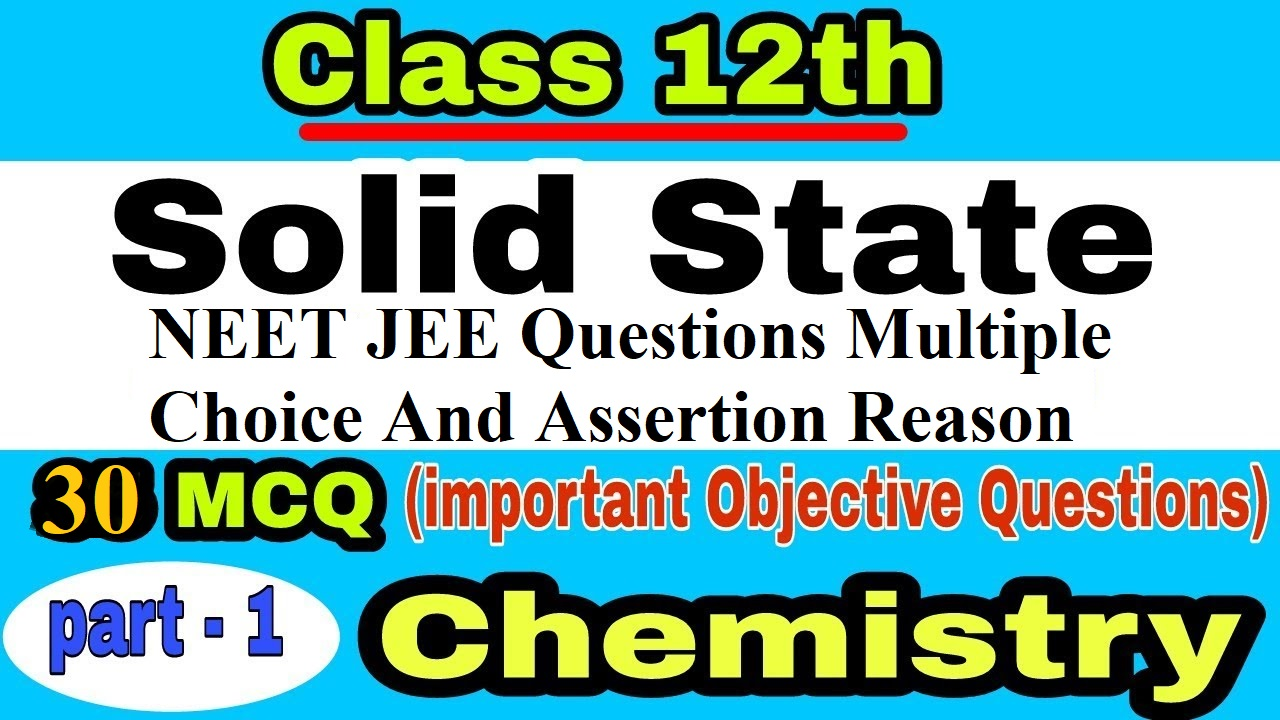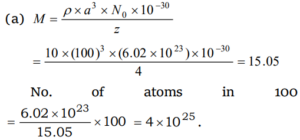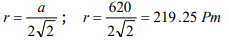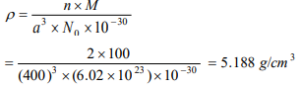# NEET JEE Solved Questions Solid State### NEET JEE Solved Questions Solid State

NEET JEE Solved Questions Solid State:

Q1. The three states of matter are solid, liquid, and gas. Which of the following statement is/are true about them                                                                                                                [AIIMS 1991]

(a) Gases and liquids have viscosity as a common property

(b) The molecules in all the three states possess random translational motion

(c) Gases cannot be converted into solids without passing through the liquid phase

(d) Solids and liquids have vapour pressure as a common property

Ans 1. (a) Both gases and liquids possess fluidity and hence viscosity molecules in the solid-state do not have translational motion.

Q2. A pure crystalline substance, on being heated gradually, first forms a turbid looking liquid, and then the turbidity completely disappears. This behaviour is the characteristic of substances forming.                                                                                                                             [BHU 2000]

(a) Isomeric crystals                                                               (b) Liquid crystals

(c) Isomorphous crystals                                                        (d) Allotropic crystals

Ans 2. (b) It is a characteristic of liquid crystal.

Q3. Which one is an example of amorphous solid

(a) Glass                                                                                  (b) Salt

(c) Cesium chloride                                                                (d) Calcium fluoride

Ans 3. (a) Amorphous solids neither have ordered arrangement (i.e. no definite shape) nor have sharp melting point like crystals, but when heated, they become pliable until they assume the properties usually related to liquids. It is therefore they are regarded as super-cooled liquids.

Q4. Silicon is                                                                                                                     [MHCET 2004]

(a) Semiconductor                                                                  (b) Insulator

(c) Conductor                                                                          (d) None of these

Ans 4. (a) Silicon is a semiconductor because it is a thermal active and its conductivity increased with increasing temperature.

Q5. Which of the following statements about amorphous solids is incorrect             [KCET 2004]

(a) They melt over a range of temperature                             (b) They are anisotropic

(c) There is no orderly arrangement of particles                    (d) They are rigid and incompressible

Ans 5. (b) Amorphous solids are isotropic, because of these substances show same properties in all directions.

Q6. Space lattice of CaF2 is                                                                                        [MP PMT 1993]

(a) Face centred cubic                                                            (b) Body centred cubic

(c) Simple cubic                                                                     (d) Hexagonal closed packing

Ans 6. (a) Space lattice of CaF2 is face centred cubic.

Q7. For cubic coordination the value of radius ratio is

(a) 0.732 -1.000                                                                     (b) 0.225 – 0.414

(c) 0.000 – 0.225                                                                    (d) 0.414 – 0.732

Ans 7. (a) For body centred cubic arrangement coordination number is 8 and radius ratio (r+/r) is 0.732 1.000.

Q8. The unit cell of a NaCl lattice

(a) Is body centred cube                                                         (b) Has 3Na+ ions

(c) Has 4NaCl units                                                                (d) Is electrically charged

Ans 8. (c) Each unit cell of NaCl contains 4 NaCl units.

Q9. A structure similar to zinc blende is found in

(a) AgCl                                                                                  (b) NaCl

(c) CuCl                                                                                  (d) TlCl

Ans 9. (c) Zinc blende (ZnS) has a fcc structure and is an ionic crystal having 4 : 4 co-ordination number.

Q10. The structure of Na2O crystal is

(a) CsCl type                                                                           (b) NaCl type

(c) ZnS type                                                                            (d) Antifluorite

Ans 10. (d) Na2O has antifluorite (A2B) type structure.

Q11. The number of tetrahedral voids in the unit cell of a face-centred cubic lattice of similar atoms is                                                                                                          [Kerala PMT 2004]

(a) 4                             (b) 6                            (c) 8                             (d) 10

Ans 11. (c) 8

Q12. The close packing represents ABC ABC…… order of

(a) Body centred cubic packing                                              (b) Face centred cubic packing

(c) Simple cubic packing                                                        (d) Hexagonal cubic closed packing

Ans 12. (b) The system ABC ABC…… is also referred to as face-centred cubic or fcc.

Q13. The arrangement ABC ABC ABC ….. is referred to as                      [MP PET 2001]

(a) Octahedral close packing                                                  (b)Hexagonal close packing

(c) Tetragonal close packing                                                  (d) Cubic close packing

Ans 13. (d) It represents ccp arrangement.

Q14. The number of close neighbour in a body-centred cubic lattice of an identical sphere is                                                                                                                          [MP PET 2001]

(a) 8                             (b) 6                            (c) 4                 (d) 2

Ans 14. (a) BCC has a coordination number of 8.

Q15. The number of equidistant oppositely charged ions in a sodium chloride crystal is                                                                                                                                      [MP PET 2001]

(a) 8                            (b) 6                            (c) 4                 (d) 2

Ans 15. (b) In rock salt structure the co-ordination number of Na+:Cl is 6 : 6.

NEET JEE Solved Questions Solid State

Q16. In the fluorite structure, the coordination number of Ca2+ ion is

(a) 4                             (b) 6                            (c) 8                 (d) 3

Ans 16. (c) CaF2 (fluorite) has fcc structure with 8 : 4 coordination number.

Q17. The ratio of close-packed atoms to tetrahedral holes in cubic close packing is [Pb. PMT 1998]

(a) 1 : 1                                   (b) 1 : 2                       (c) 1 : 3                       (d) 2 : 1

Ans 17. (b) Every constituent has two tetrahedral voids. In ccp lattice atoms

= 8×1/8 + 6×1/2 = 4

Tetrahedral void = 4 × 2 = 8

Q18. Potassium fluoride has NaCl type structure. What is the distance between K+ and F ions if cell edge is a cm

(a) 2a cm                     (b) a / 2 cm                  (c) 4a cm         (d) a / 4 cm

Ans 18. (b) Distance between K+ and F = ½ × length of the edge

Q19. An element occurring in the bcc structure has 12.08 ´1023unit cells. The total number of atoms of the element in these cells will be                                                       [MP PET 1994]

(a) 24.16 × 1023                                                                                    (b) 36.18 × 1023

(c) 6.04 × 1023                                                                                       (d) 12.08 ×1023

Ans 19. (a) There are two atoms in a bcc unit cell. So, number of atoms in 12.08 × 1023 unit cells = 2 × 12.08 × 1023 atom  = 24.16 × 1023.

Q20. For an ionic crystal of the general formula AX and coordination number 6, the value of radius ratio will be                                                                                                    [MP PMT 1993]

(a) Greater than 0.73                                                               (b) In between 0.73 and 0.41

(c) In between 0.41 and 0.22                                                  (d) Less than 0.22

Ans 20. (b) The structural arrangement of co-ordination number ‘6’ is octahedral and its radius ratio is 0.414 0.732. The example of octahedral is KCl and NaCl.

Q21. The number of atoms in 100 g of an fcc crystal with density d = 10 g / cm3 and cell edge equal to 100 pm, is equal to                                                                          [CBSE PMT 1994; KCET 2002]

(a) 4 × 1025                  (b) 3 × 1025                  (c) 2 × 1025                   (d) 1 × 1025

Ans 21. (a)Q22. The number of unit cells in 58.5 g of NaCl is nearly                        [MP PMT 2000, 01]

(a) 6 × 1020                   (b) 3 × 1022                  (c) 1.5 × 1023                (d) 0.5 × 1024

Ans 22. (c) 58.5 g NaCl =1 mole = 6 × 1023 Na+Cl units.

One-unit cell contains 4 Na+ Cl units. Hence number of unit cell present

= 6.021 ×1023/4 = 1.5 × 1023.

Q23. The edge of the unit cell of FCC Xe crystal is 620 pm. The radius of the Xe atom is                                                                                                                                                       [MP PET 2004]

(a) 219.25 Pm             (b) 235.16 Pm                         (c) 189.37 Pm             (d) 209.87 Pm

Ans 23.  (a)Q24. Potassium crystallizes in a bcc lattice, hence the coordination number of potassium in potassium metal is                                                                                                               [KCEE 1993]

(a) 0                           b) 4                              (c) 6                             (d) 8

Ans 24. (d) For bcc lattice, co-ordination number is 8.

Q25. Body centred cubic lattice has a coordination number of                                                                                                                                                      [AIIMS 1996; MP PMT 2002]

(a) 4                             (b) 8                            (c) 12                           (d) 6

Ans 25. (b) Body centered cubic lattice has a co-ordination number 8.

Q26. How many chloride ions are there around sodium ion in sodium chloride crystal

[NCERT 1979, 80; CPMT 1988; BHU 1982, 87; MP PET 1995, 99]

(a) 3                             (b) 8                            (c) 4                             (d) 6

Ans 26. (d) In NaCl crystal every Na+ ion is surrounded by 6 Cl ion and every chloride ion is surrounded by 6 Na+ ions.

NEET JEE Solved Questions Solid State

Q27. Which of the following statement(s) is(are) correct                                                    [IIT 1998]

(a) The coordination number of each type of ion in CsCl crystal is 8

(b) A metal that crystallizes in bcc structure has a coordination number of 12

(c) A unit cell of an ionic crystal shares some of its ions with other unit cells

(d) The length of the unit cell in NaCl is 552 pm (rNa+ = 95 pm; rCl- = 181 pm)

Ans 27. (acd) A metal that crystallizes in bcc structure has a co-ordination number of 8.

Q28. Schottky defect generally appears in                                                             [DCE 2004]

(a) NaCl                      (b) KCl                                    (c) CsCl                                   (d) All of these

Ans 28. (d) Schottky defects occurs in highly ionic compounds which have high co-ordination number ex. NaCl, KCl, CsCl.

Q29. Due to Frenkel defect, the density of ionic solids                 [MP PET 1996; MP PMT 2002]

(a) Increases                (b) Decreases              (c) Does not change    (d) Changes

Ans 29. (c) Since no ions are missing from the crystal as a whole, there is no effect on density.

Q30. An element (atomic mass 100 g / mol) having bcc structure has unit cell edge 400 pm. Then density of the element is                                                        [CBSE PMT 1996; AIIMS 2002]

(a) 10.376 g / cm3       (b) 5.188 g / cm3         (c) 7.289 g / cm3         (d) 2.144 g / cm3

Ans 30. (b)Assertion and Reason Type

Read the assertion and reason carefully to mark the correct option out of the options given below:

(a) If both assertion and reason are true and the reason is the correct explanation of the assertion.

(b) If both assertion and reason are true but the reason is not the correct explanation of the assertion.

(c) If assertion is true but reason is false.

(d) If the assertion and reason both are false.

(e) If assertion is false but reason is true.

Q31. Assertion: Diamond is a precious stone.

Reason: Carbon atoms are tetrahedrally arranged in diamond.                                [AIIMS 1994]

Ans 31. (b) It is true that in the diamond structure, carbon atoms are arranged in tetrahedrally (sp3 hybridized) but it is not the correct explanation of assertion.

Q32. Assertion: In the crystal lattice, the size of the cation is larger in a tetrahedral hole than in an octahedral hole.

Reason: The cations occupy more space than anions in crystal packing.                 [AIIMS 1996]

Ans 32. (d) Tetrahedral holes are smaller in size than octahedral holes. Cations usually occupy less space than anions.

Q33. Assertion: Crystalline solids have short-range order.

Reason: Amorphous solids have long-range order.                                                   [AIIMS 1999]

Ans 33. (d) Crystalline solids have a regular arrangement of constituent particles and are anisotropic whereas amorphous solids have no regular arrangement and are isotropic.

Q34. Assertion: In an ionic solid (MX) with Schottky defects, the number of positive and negative ions are the same.

Reason: Equal number of cation and anion vacancies are present.               [IIT Screening 2001]

Ans 34. (a) The Schottky defect is due to missing of an equal number of cations and anions.

Q35. Assertion: Space or crystal lattice differ in symmetry of the arrangement of points.

Reason: nl = 2d sinq , is known as Bragg’s equation.

Ans 35. (b) Space or crystal lattice is a regular repeating arrangement of points in space and forms the basis of classification of all structures.

Q36. Assertion: In close packing of spheres, a tetrahedral void is surrounded by four spheres whereas an octahedral void is surrounded by six spheres.

Reason: A tetrahedral void has a tetrahedral shape whereas an octahedral void has an octahedral shape.

Ans 36. (c) The tetrahedral void is so called because it is surrounded by four spheres tetrahedrally while octahedral void is so called because it is surrounded by six spheres octahedrally.

Q37. Assertion: Cyclic silicates and chain silicates have the same general molecular formula.

Reason: In cyclic silicates, three corners of each SiO4 tetrahedron are shared while in chain silicates only two are shared with other tetrahedra.

Ans 37. (c) Two corners per tetrahedron one shared in both the cases.

Q38. Assertion: The presence of a large number of Schottky defects in NaCl lowers its density.

Reason: In NaCl, there are approximately 106 Schottky pairs per 3 cm at room temperature.

Ans 38. (b) When an atom or an ion is missing from its normal lattice site, a lattice vacancy or defect is created, which is called Schottky defect. Due to the missing density of crystal will be lowered.

Q39. Assertion: Anion vacancies in alkali halides are produced by heating the alkali halide crystals with alkali metal vapour.

Reason: Electrons trapped in anion vacancies are referred to as F -centres.

Ans 39. (b) On heating, the metal atoms deposit on the surface and finally they deffuse into the crystal and after ionisation the alkali metal ion occupies cationic vacancy where as electron occupies anionic vacancy.

Q40. Assertion: Electrical conductivity of semiconductors increases with increasing temperature.

Reason: With an increase in temperature, a large number of electrons from the valence band can jump to the conduction band.

Ans 40. (a) In case of semiconductors, the gap between the valence band and the conduction band is small and therefore some of the electrons may jump from valence band to conduction band and thus on increasing temperature conductivity is also increased.

Q41. Assertion: On heating ferromagnetic or ferrimagnetic substances, they become paramagnetic.

Reason: The electrons change their spin on heating.

Ans 41. (a) All magnetically ordered solids (ferromagnetic and antiferromagnetic solids) transform to the paramagnetic state at high temperature due to the randomisation of spins.

Q42. Assertion: Lead zirconate is a piezoelectric crystal.

Reason: Lead zirconate crystals have no dipole moment.

Ans 42. (c) In piezoelectric crystals, the dipoles may align themselves in an ordered manner such that there is a net dipole moment in the crystal.

NEET JEE Solved Questions Solid State